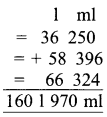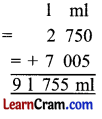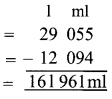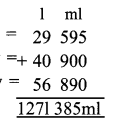# DAV Class 4 Maths Chapter 7 Worksheet 4 Solutions

The DAV Class 4 Maths Solutions and DAV Class 4 Maths Chapter 7 Worksheet 4 Solutions of Capacity offer comprehensive answers to textbook questions.

## DAV Class 4 Maths Ch 7 WS 4 Solutions

Question 1.
Solve the following word problems:
(a) Three milkmen are separately carrying 36 1 250 ml, 58 1 396 ml and 66 1 324 ml of milk in their containers. Find out what is the total quantity of milk with them.
Quantity of milk in one container = 36 l 250ml
Quantity of milk in 2nd container = 58l 396ml
Quantity of milk in 3rd container = 66l 324ml
Total milk in containers = 160 1 970 ml160 1 970 ml.

(b) A shopkeeper purchased 45 1 500 ml of mineral water. During the day, he sold out 28 1 755 ml water. How much mineral water was left with him?
Total quantity of mineral water purchasedQuantity of mineral water sold out = 28 755
Mineral water left = 161 745ml
16 1 745 ml.

(c) Raju mixes 2 1 750 ml of cow’s milk in 7 1 5 ml of buffalo’s milk. How much milk did Raju have in all?
Quantity of cow’s milk Raju has = 2l 750ml
Quantity of buffalo’s milk Raju has = 7l 005ml
Total quantity of milk Raju has = 9 l 755 ml9 l 755 ml.(d) A barrel can hold 29 1 55 ml of oil. 12 1 94 ml oil was taken out. What is the remaining quantity of oil in the barrel?
Capacity of barrel = 29l 055ml
Quantity of oil taken out = – 12l 094ml
Oil left in barrel = 161 961ml16 l 961 ml.

(e) Ramu’s bucket holds 9 1 350 ml of water and Shalu’s bucket holds 8 1 455 ml of water. Whose bucket holds more water and by how much?
As 9 1 350 ml > 8 1 455 ml
Ramu’s bucket holds more water.
Ramu’s bucket hold = 9l 350ml
Shalu’s bucket hold = 8l 455ml
Difference = 01 895 ml895 ml of water.

### DAV Class 4 Maths Chapter 7 Value Based Questions

Question 1.
How much water was wasted due to leakage in the tanker?
Water provided = 15000l 000ml
Water received = – 13200l 500ml
Water wasted = 1799l 500 ml1799l 500 ml of water.

Question 2.
Suggest any two ways by which you can avoid wastage of water in your house.
1. Stop leakage of taps in the house.
2. Switch off water motor immediately after the filling of tank.

Word Problems
Question 1.
A milkman sold 29l 595 ml of milk on the first day, 40 1 900 ml on the second day and 56l 890 ml milk on the third day. What is the total quantity of milk sold on three days?
We add all the quantities to get the total quantity.
Quantity of milk sold on 1st day = 29l 595ml
Quantity of milk sold on 2nd day = 40l 900ml
Quantity of milk sold on 3rd day = 56l 890ml
Total quantity of milk sold = 127l 385ml127 l 385 ml.Question 2.
There was 5 1 890 ml of oil in a can. Rosy used 2l 750 ml of oil for cooking. How much oil is left in the can?